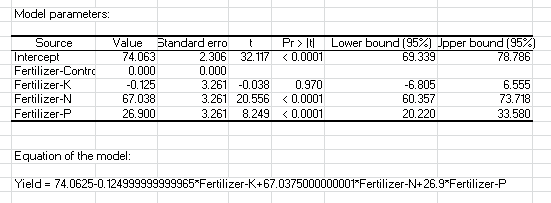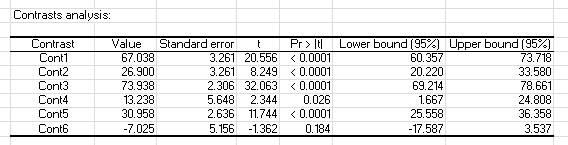# Contrast analysis after a one-way ANOVA in Excel

2017-10-20

This tutorial will help you set up and interpret contrasts following a one-way Analysis of Variance (ANOVA) in Excel using the XLSTAT software.

## Dataset for running contrast analysis following a one-way ANOVA

An Excel sheet with both the data and the results can be downloaded by clicking on the button below:

The data correspond to an agronomy experiment aiming at testing the effects of four treatments (control, K, N and P fertilizers) on corn yield. Every treatment is applied inside eight distinct plots.

## Goal of this tutorial

The aim of this tutorial is to answer very specific questions we may ask after running a linear model (one-way ANOVA in our case) using contrast analysis. For example, we may ask if one fertilizer is able to significantly increase the corn yield two or three-fold. We may also test the significance of the difference between two distinct groups of means (do the three fertilizers significantly increase the yield in average compared to the control yield?). It is possible to address these questions by using contrast analysis. Technically, a contrast is a weighted sum of the coefficients of a linear model. We test the difference between this sum and zero.

## A few preliminary steps

Before illustrating the concept of contrasts and proceeding with the analyses, we strongly recommend extracting the names of the linear model coefficients (one-way ANOVA in our case) as they are provided by XLSTAT. Those names may easily be taken out in the result sheet produced by a classical ANOVA analysis in XLSTAT. Select the XLSTAT / Modeling data / ANOVA command, or click on the corresponding button of the Modeling Data toolbar. This tutorial may help you in launching the analysis. Configuration: dependent variable = Yield; explanatory variable = Fertilizer.

In the results sheet, take a look at the model parameters table.The first column (Source) contains the names of the model’s coefficients as defined by XLSTAT. The second column (Value) includes coefficient values structuring the model’s equation below, linking the explanatory variable (“Fertilizer” factor) to the dependent variable (Yield). By default, XLSTAT uses the mean of the first level (in alphanumeric order) of the studied factor as a reference level (Intercept). In our data, this level corresponds to the Control. The effects are calculated as deviations between the other levels means and the reference level mean. For example, the deviation between P and control is 26.90. It is significantly different from zero at the risk alfa = 0.05 (P-value < 0.0001).

Notice that effect computation type may be modified in XLSTAT in the ANOVA command / Options tab / Constraints. An an=0 constraint will use the last level (P in our case) as a reference. A sum(ai)=0 constraint will compute the differences between all levels and the general mean of the data. The less-frequently used sum(ni.ai)=0 constraint allows in addition to take group size into account.## Contrast analysis, a conceptual approach

Conceptually, in order to test if a coefficient value is significantly different from zero, we may set all the other coefficients in the model’s equation to zero and compare the resulting value to zero.

For example, if we are interested by the FertilizerN coefficient, the model’s equation would be written this way:

0 x Intercept + 0 x FertilizerC + 0 x FertilizerK + FertilizerN + 0 x FertilizerP                             (1)

= FertilizerN

= 67.038

This value is tested against zero and is significant in our case (P value < 0.0001).

Consider equation (1) and multiply FertilizerN by 1:

0 x Intercept + 0 x FertilizerC + 0 x FertilizerK + 1 x FertilizerN + 0 x FertilizerP                       (1)

The combination of numbers multiplying every coefficient in a particular test is a contrast. Here, we arbitrarily call our contrast Cont1, and we write it the following way:

Cont1: 0 0 0 1 0

The contrast testing the FertilizerP coefficient may be defined as follows:

Cont2: 0 0 0 0 1

We may define a contrast to test if the total yield of the K-fertilized plots is significantly different from zero. Therefore we compute the sum between the reference level (Intercept) and the deviation explained by fertilizer K (FertilizerK coefficient) and to test if this sum is significantly different from zero. The contrast is written as follows:

Cont3: 1 0 1 0 0

We may ask if the N fertilizer yield improvement is at least twice as important as the improvement provided by the P fertilizer. This is represented by a positive and significant FertilizerN – 2 x FertilizerK difference. The following contrast translates this difference:

Cont4: 0 0 0 1 -2

Furthermore, we may be interested by the average yield improvement provided by the three fertilizers (K, N and P) compared to the control yield. This question may be addressed by calculating the average between fertilizer deviations and to test its significance. Mathematically, this average is written as follows:

(FertilizerK + FertilizerN + FertilizerP)/3 = 0.33 x FertilizerK + 0.33 x FertilizerN + 0.33 x FertilizerP

The equivalent contrast:

Cont5: 0 0 0.33 0.33 0.33

Finally, we may ask if the N fertilizer increases the control yield at least twice-fold. Mathematically, this may be tested by checking if the value defined by the difference between the average yield of the N-fertilized plots and twice the average yield of the control plots is positive and significant. Using model coefficients, this may be translated as follows:

Average(N-fertilized plots) – 2 x average(Control plots)

= Intercept + FertilizerN – 2 x (Intercept + FertilizerControl)

= FertilizerN – Intercept

The equivalent contrast:

Cont6: -1 0 0 1 0

## How to do it in XLSTAT

In the ANOVA results sheet, copy the column containing coefficient names and paste it in the data sheet. This step is not mandatory but makes contrast definition easier.

Write the desired contrasts in columns next to the coefficients (one column per contrast). In this tutorial, we consider the 6 contrasts defined above.

For a better readability, include contrast names above contrast columns.Configure the same ANOVA described above, adding options under the Output / Contrasts tab: tick the Compute contrasts box and select the contrast matrix including column names but excluding coefficients. Click on the OK button.

The contrasts analysis table appears at the bottom of the results sheet.Every contrast is defined by a value corresponding to the tested quantity which was calculated in the contrast’s equation. This quantity is associated to a standard error and to lower and upper boundaries of a 95% confidence interval. Finally, a t statistic associated to a p-value allows testing if the quantity is significantly different from zero.

Here is a summary of the questions we asked in the above paragraph and the answers we provide through contrast analysis:

Cont1: Is the deviation between the N fertilizer and the control is significant at alpha = 0.05?

Cont2: Is the deviation between the P fertilizer and the control is significant at alpha = 0.05?

Cont3: What is the mean yield of plots submitted to a K fertilizer? Is this yield significantly different from zero at alpha = 0.05?

Answer: mean yield = 73.938, significantly different from zero (P-value < 0.0001).

Cont4: Is the N yield improvement at least twice as important as the P improvement at alpha = 0.05?

Answer: yes because the resulting contrast value is positive (+13.238) and significant (P-value <0.05).

Cont5: what is the average yield improvement provided by the three fertilizers (K, N and P) compared to the control yield? Is this improvement significant at alpha = 0.05?

Answer: improvement = 30.958, significantly different from zero (P-value < 0.0001).

Cont6: Does the N fertilizer increase at least twice-fold the total yield compared to the control yield at alpha = 0.05?

Answer: the difference between the yield of the N-fertilized plots and twice the yield of the control plots is not significant (P-value > 0.05).

### Going further

Contrasts may be defined on linear models of varying complexity (e.g. multi-level ANOVA with interactions, ANCOVA, etc.). In models including quantitative explanatory variables, it is thus possible to manipulate coefficients corresponding to slopes or to slope deviations.

#### Contact our technical support team: support@xlstat.com

https://cdn.desk.com/
false
desk